# Identify the isoprene units in the following terpenes. Classify each terpene based on the number of...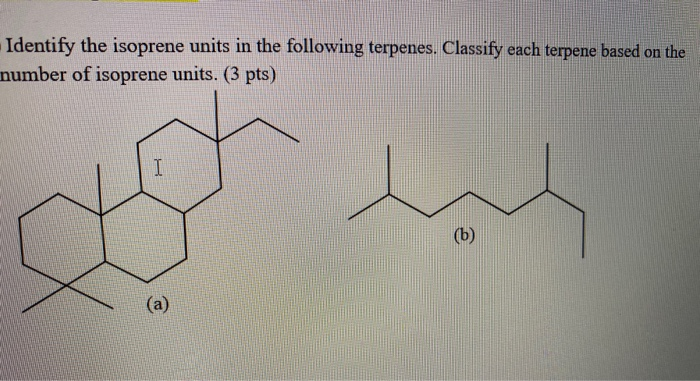Identify the isoprene units in the following terpenes. Classify each terpene based on the number of isoprene units. (3 pts) I (6) (a)
Show transcribed image text

This Homework Help Question: "Identify the isoprene units in the following terpenes. Classify each terpene based on the number of..." No answers yet.

We need 10 more requests to produce the answer to this homework help question. Share with your friends to get the answer faster!

0 /10 have requested the answer to this homework help question.

Once 10 people have made a request, the answer to this question will be available in 1-2 days.
All students who have requested the answer will be notified once they are available.
##### Add Answer of: Identify the isoprene units in the following terpenes. Classify each terpene based on the number of...
Similar Homework Help Questions
• ### Question2. Show the isoprene units in each of the following terpenes and classify them. HC CH,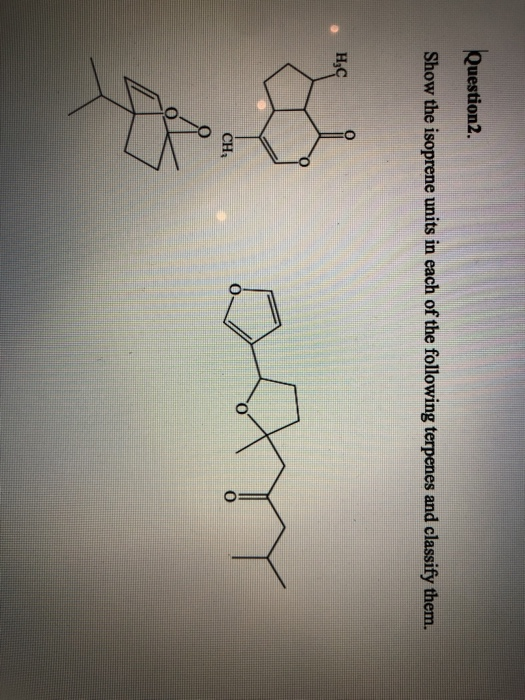Question2. Show the isoprene units in each of the following terpenes and classify them. HC CH,

• ### Show (circle) the positions of the isoprene units in each of the indicated terpenes. (5 marks)...

Show (circle) the positions of the isoprene units in each of the indicated terpenes. (5 marks)                       β-Selinine                                                            Grandisol

• ### Q3 case, do SO. (2 points) ruarus: ulat Is the How many isoprene units are in...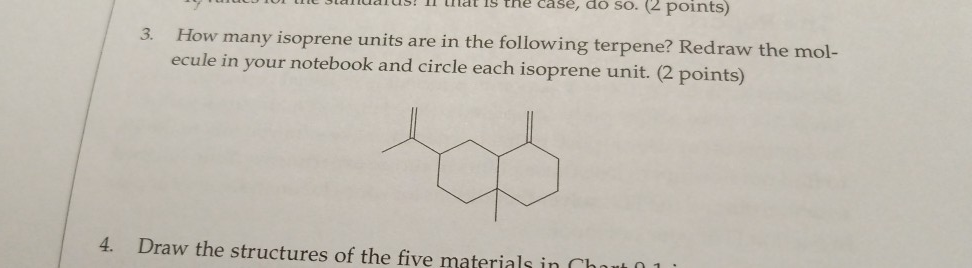Q3 case, do SO. (2 points) ruarus: ulat Is the How many isoprene units are in the following terpene? Redraw the mol- ecule in your notebook and circle each isoprene unit. (2 points) 3. 4. Draw the structures of the five materials in Cha

• ### 6. Classify each of the following solids according to the forces of attraction that exist between...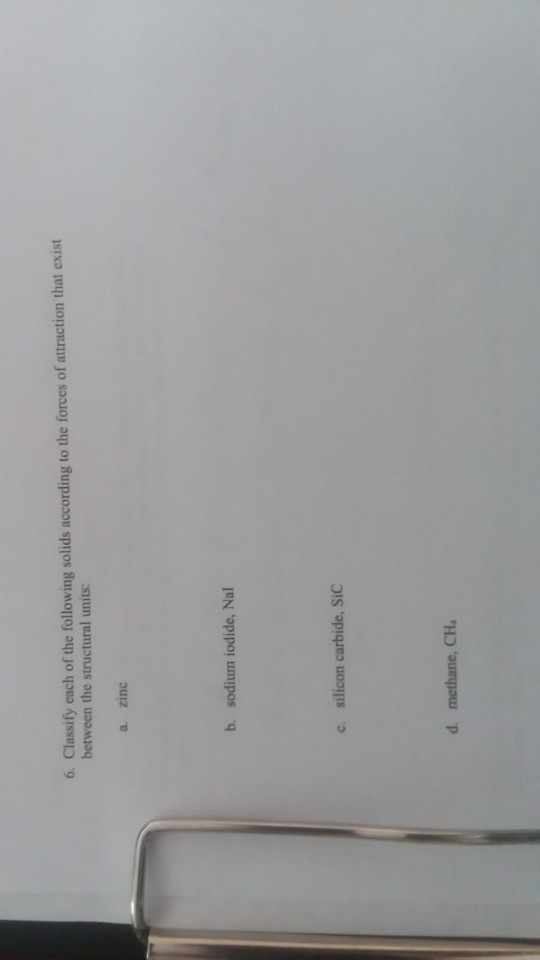6. Classify each of the following solids according to the forces of attraction that exist between the structural units: a zinc b. sodium iodide, Nal csilicon carbide, SIC d. methane, CH Show transcribed image text

• ### QUESTION 4 Based on your answers to question 2 and 3 identify each of the following...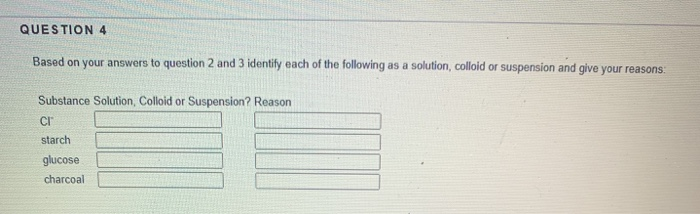QUESTION 4 Based on your answers to question 2 and 3 identify each of the following as a solution, colloid or suspension and give your reasons: Substance Solution Colloid or Suspension? Reason CI starch glucose charcoal Show transcribed image text

• ### Question 4 3 pts Classify the following Amines as Primary, Secondary, and Tertiary. a) NH2 b)...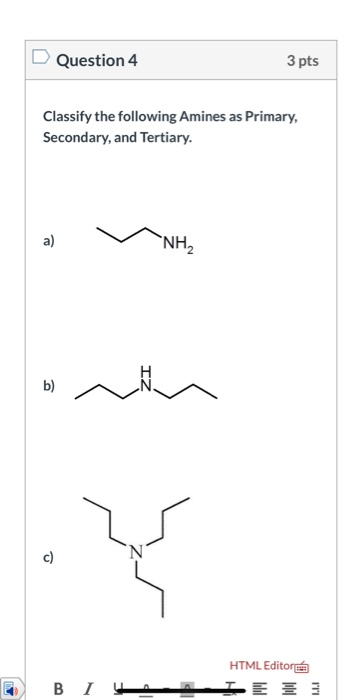Question 4 3 pts Classify the following Amines as Primary, Secondary, and Tertiary. a) NH2 b) c) HTML Editora B I VAAIETE Show transcribed image text

• ### Question 9 3 pts Classify each of the following substances as acid (A), base (B) or...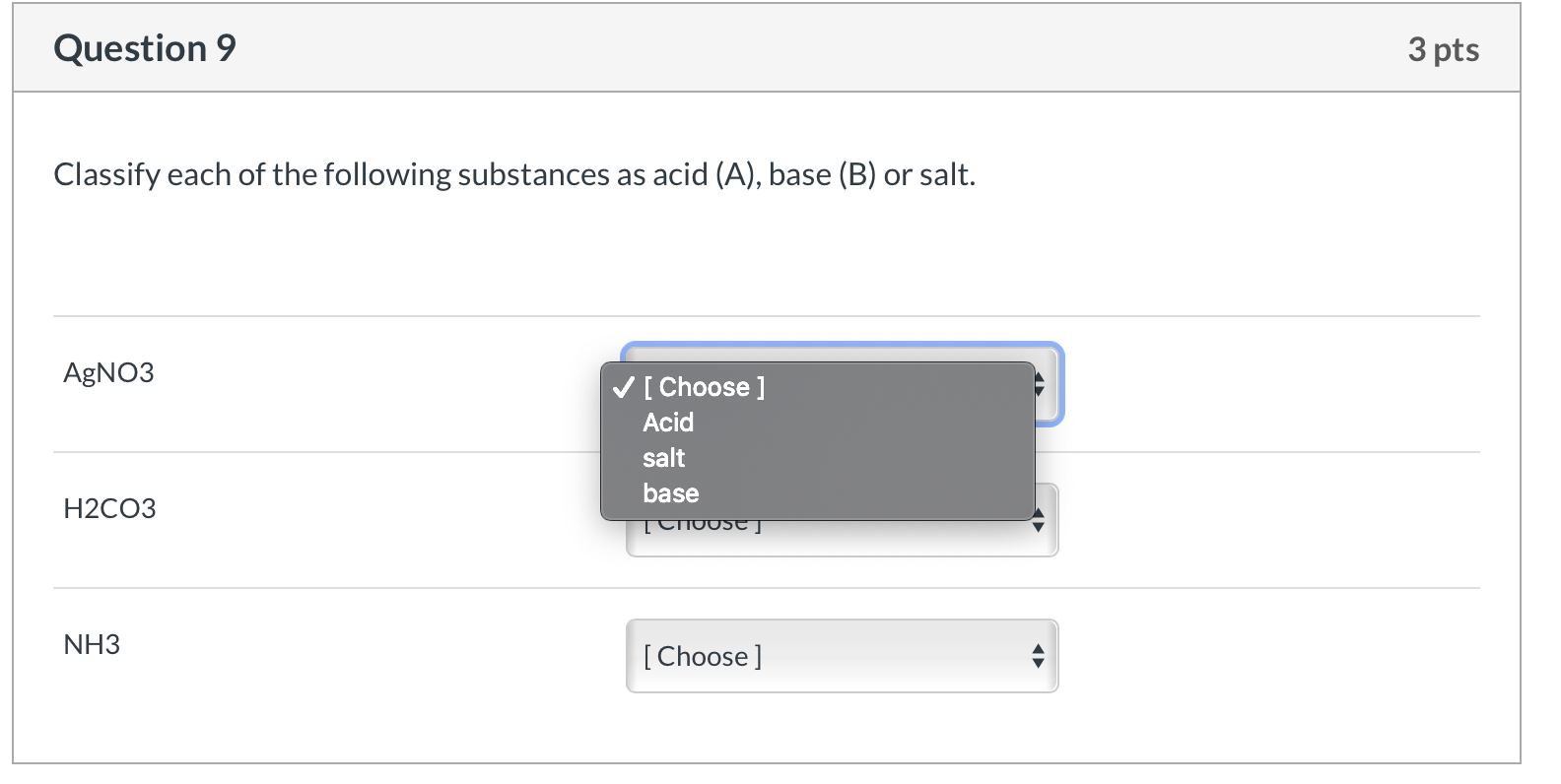Question 9 3 pts Classify each of the following substances as acid (A), base (B) or salt. AgNO3 [Choose ] Acid salt base H2CO3 TCIOUSC] NH3 [Choose] Question 13 7 pts Balance the following chemical equations. If you leave a space blank, I will assume the coefficient is “1”, or you can write in a 1 on the line. Al2(SO4)3 + BaCl2 → _AlCl3 + BaSO4 Co + H2O → _C0203 + _H2 For clarity Co is Cobalt Question 12...

• ### D Question 6 6 pts Classify the following compounds as soluble or insoluble in water. Explain...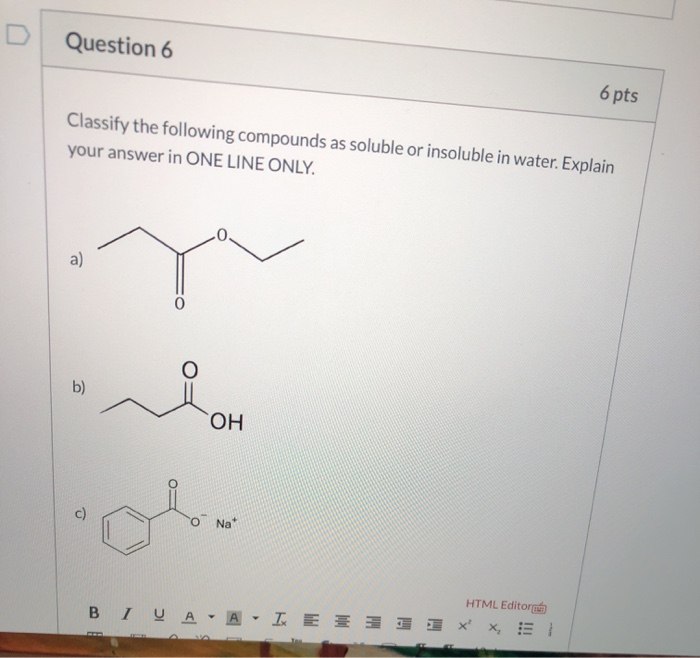D Question 6 6 pts Classify the following compounds as soluble or insoluble in water. Explain your answer in ONE LINE ONLY. 0. a) Y b) OH Na HTML Editor B IV A - A - IE * 3 1 1 x X. !! Show transcribed image text

• ### In an atom, identify the number of electrons that could be characterized by the following, N=...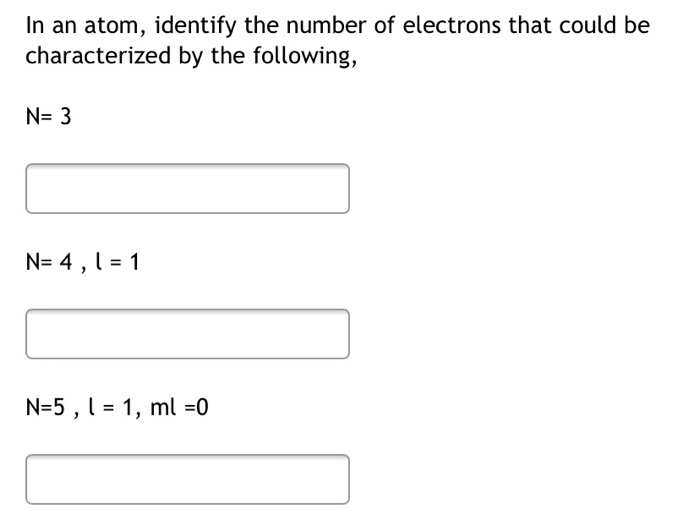In an atom, identify the number of electrons that could be characterized by the following, N= 3 N= 4,1 = 1 N=5 , 1 = 1, ml =0 Show transcribed image text

• ### S.4. Classify each carbon atom as primary, secondary, and tertiary in the following molecules. X a)...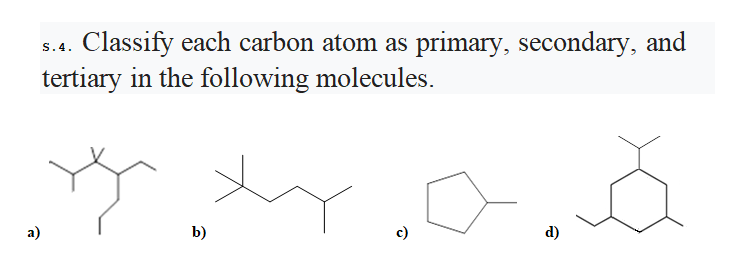S.4. Classify each carbon atom as primary, secondary, and tertiary in the following molecules. X a) b) c) d) Show transcribed image text

Free Homework App